# NCERT Solutions for Class 9 Math Chapter 7 - Triangles

##### Question 1:

In quadrilateral ACBD, AC = AD and AB bisects ∠A (see fig.). Show that $∆$ABC $\cong$ $∆$ABD.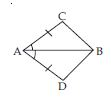What can you say about BC and BD?

AC = AD and AB bisects ∠A.
We have to prove that $∆$ABC $\cong$ $∆$ABD.
Proof: In $∆$ABC and $∆$ABD,
∠BAC = ∠BAD [$\because$ AB bisects ∠A (given)]
AB = AB (common)
$\therefore$ $∆$ABC $\cong$ $∆$ABD [SAS criteria of congruency]
Thus BC = BD
(Congruent parts of congruent triangles)

##### Question 2:

and ∠DAB = ∠CBA (see fig.). Prove that
(i ) $∆$ABD $\cong$ $∆$BAC
(ii) BD = AC
(iii) ∠ABD = ∠BAC.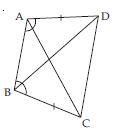In $∆$ABD and $∆$ABC,
∠DAB = ∠CBA (given)
AB = AB (common)
(i ) $∆$ABD $\cong$ $∆$BAC
(SAS criteria of congruency)
(ii) BD = AC
(Congruent parts of congruent triangles)
(iii) ∠ABD = ∠BAC
(Congruent parts of congruent triangles)

##### Question 3:

AD and BC are equal perpendiculars to a line segment AB (see fig.). Show that CD bisects AB.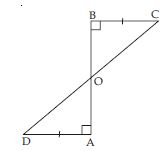In $∆$BOC and $∆$AOD,
[each 90$°$ (given)]
∠BOC = ∠AOD
(vertically opposite angles)
$\therefore$ $∆$BOC $\cong$ $∆$AOD
(AAS criteria of congruency)
$⇒$ OB = OA
and OC = OD
(Congruent parts of congruent triangles)
It implies that O is the mid-point of line-segment AB and CD.

##### Question 4:

l and m are two parallel lines intersected by another pair of parallel lines p and q (see fig.) Show that $∆$ABC $\cong$ $∆$CDA.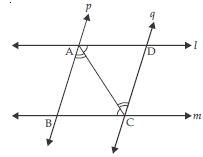Given than l || m
AC being a transversal.
Therefore ∠ACB = ∠DAC (alternate angles)
p || q (given)
AC being a transversal.
Therefore∠BAC = ∠ACD (alternate angles)
Now in $∆$ABC and $∆$CDA,
∠ACB = ∠DAC (Proved above)
∠BAC = ∠ACD (Proved above)
AC = AC (Common)
$\therefore$ $∆$ABC $\cong$ $∆$CDA
(AAS criteria of congruency)

##### Question 5:

Line l is the bisector of an angle ∠A and B is any point on l. BP and BQ are perpendiculars from B to the arms of ∠A (see fig.). Show that:
(i) $∆$APB $\cong$ $∆$AQB
(ii) BP = BQ or B is equidistant from the arms of ∠A.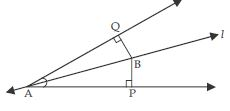Given that line l bisects ∠A.
$\therefore$ ∠BAP = ∠BAQ
Now in $∆$APB and $∆$AQB,
∠BAP = ∠BAQ (given)
∠BPA = ∠BQA [each 90$°$ (given)]
AB = AB (common)
$\therefore$ $∆$APB $\cong$ $∆$AQB
(AAS criteria of congruency)
$⇒$ BP = BQ
Congruent parts of congruent triangles i.e., B is
equidistant from the arms of ∠A.

##### Question 6:

In fig., AC = AE, AB = AD and ∠BAD = ∠EAC. Show that BC = DE.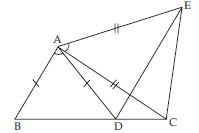Given that
Adding ∠DAC on both sides, we get
∠BAD + ∠DAC = ∠EAC + ∠DAC
$⇒$ ∠BAC = ∠EAD ... (i)
Now in $∆$ABC and $∆$AED,
AC = AE (given)
$\therefore$ $∆$ABC $\cong$ $∆$AED
(AAS criteria of congruency)
$⇒$ BC = DE
(Congruent parts of Congruent triangles)

##### Question 7:

AB is a line segment and P is its mid-point. D and E are points on the same side of AB such that ∠BAD = ∠ABE and ∠EPA = ∠DPB (see fig.). Show that
(i) $∆$DAP $\cong$ $∆$EBP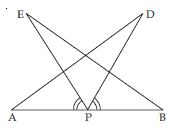Given that
∠EPA = ∠DPB
Adding ∠EPD on both sides, we get:
∠EPA + ∠EPD = ∠DPB + ∠EPD
$⇒$ ∠APD = ∠BPE ... (i)
Now in $∆$DPA and $∆$EBP,
∠PAD = ∠PBE [$\because$ ∠BAD = ∠ABE
(given) $\therefore$ ∠PAD = ∠PBE]
∠APD = ∠BPE [From (i)]
AP = PB
[$\because$ P is the mid-point of AB (given)]
$\therefore$ $∆$DPA $\cong$ $∆$EBP
[AAS criteria of congruency]
$⇒$ AD = BE
(Congruent parts of congruent triangles)

##### Question 8:

In right triangle ABC, right angled at C, M is the mid-point of hypotenuse AB. C is joined to M and produced to a point D such that DM = CM. Point D is joined to point B (see fig.). Show that: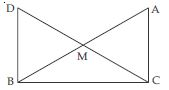$∆$AMC $\cong$ $∆$BMD

In $∆$AMC and $∆$BMD,
AM = BM
[$\because$ M is the mid-point of hyp. AB (given)]
∠AMC = ∠BMD
(vertically opp. angles)
CM = DM (given)
$\therefore$ $∆$AMC $\cong$ $∆$BMD
(SAS criteria of congruency)
$\therefore$ ∠ACM = ∠BDM ... (a)
∠CAM = ∠DBM
and AC = BD (Congruent parts of Congruent triangles)

##### Question 9:

In right triangle ABC, right angled at C, M is the mid-point of hypotenuse AB. C is joined to M and produced to a point D such that DM = CM. Point D is joined to point B (see fig.). Show that:∠DBC is a right angle.

For two lines AC and DB and transversal DC,
we have
∠ACD = ∠BDC (alternate angles)
[$\because$ ∠ACM = ∠BDM using (a)
$\therefore$ ∠ACD = ∠BDC]
$\therefore$ AC || DB [$\because$ If alternate angles are equal then lines are parallel]
Now for parallel lines AC and DB and for transversal BC.
∠DBC = ∠ACB (alternate angles)
... (b)
But $∆$ABC is a rt. ∠d triangle, right angled at C.
$\therefore$ ∠ACB = 90$°$ ... (c)
Therefore ∠DBC = 90$°$ [Using (b) and (c)]
It implies that ∠DBC is a right triangle.

##### Question 10:

In right triangle ABC, right angled at C, M is the mid-point of hypotenuse AB. C is joined to M and produced to a point D such that DM = CM. Point D is joined to point B (see fig.). Show that:$∆$DBC $\cong$ $∆$ACB

Now in $∆$DBC and $∆$ACB,
DB = AC [Proved in part (i)]
∠DBC = ∠ACB
[each 90$°$ proved in part (ii)]
BC = BC (common)
$\therefore$ $∆$DBC $\cong$ $∆$ACB
(SAS criteria of congruency)

##### Question 11:

In right triangle ABC, right angled at C, M is the mid-point of hypotenuse AB. C is joined to M and produced to a point D such that DM = CM. Point D is joined to point B (see fig.). Show that: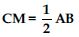In part (iii) we have proved that
$∆$DBC $\cong$ $∆$ACB
$\therefore$ DC = AB
$⇒$DM + CM = AB
$⇒$CM + CM = AB [$\because$ DM = CM (given)]
$⇒$ 2 CM = AB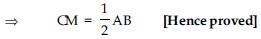##### Question 12:

In an isosceles triangle ABC, with AB = AC, the bisectors of ∠B and ∠C intersect each other at O. Join A to O show that:
OB = OC

ABC is an isosceles triangle in which AB = AC
$\therefore$ ∠C = ∠B
(angles opposite to equal sides)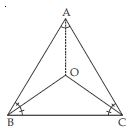$⇒$ ∠OCA + ∠OCB = ∠OBA + ∠OBC
$⇒$ ∠OCB + ∠OCB = ∠OBC + ∠OBC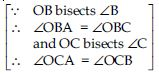$⇒$ 2∠OCB = 2∠OBC
$⇒$ ∠OCB = ∠OBC
Now in $∆$OBC,
∠OCB = ∠OBC (proved above)
$\therefore$ OB = OC (sides opposite to equal angles)

##### Question 13:

In an isosceles triangle ABC, with AB = AC, the bisectors of ∠B and ∠C intersect each other at O. Join A to O show that:
AO bisects ∠A.

Now in $∆$AOB and $∆$AOC
AB = AC (given)
∠OBA = ∠OCA
$\because$ ∠B = ∠C
BO bisects ∠B and CO bisects ∠C.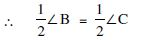$⇒$ ∠OBA = ∠OCA
AO = AO (Common)
$\therefore$ $∆$AOB $\cong$ $∆$AOC
(SAS criteria of congruency)
$⇒$ ∠OAB = ∠OAC
(Congruent parts of congruent triangles)
Hence AO; bisects ∠A.

##### Question 14:

In $∆$ABC, AD is the perpendicular bisector of BC (see fig.). Show that $∆$ABC is an isosceles triangle in which AB = AC.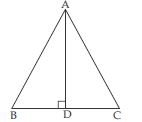In $∆$ABD and $∆$ACD,
BD = CD
($\because$ AD bisects BC) [given]
= each 90$°$ ($\because$ AD $\perp$ BC) [given]
$\therefore$ $∆$ABD $\cong$ $∆$ACD
(SAS criteria of congruency)
$⇒$ AB = AC
(Congruent parts of congruent triangles)
Therefore ABC is an isosceles triangle.

##### Question 15:

ABC is an isosceles triangle in which altitudes BE and CF are drawn to sides AC and AB respectively (see fig.). Show that these altitudes are equal.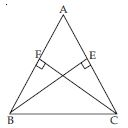In $∆$ABE and $∆$ACF,
∠A = ∠A [Common]
∠AEB = ∠AFC (each = 90$°$) [given]
AB = AC [given]
$\therefore$ $∆$ABE $\cong$ $∆$ACF
(AAS criteria of congruency)
Therefore
BE = CF
(Congruent parts of congruent triangles)
In other words, altitudes drawn to respective equal sides are equal.

##### Question 16:

ABC is a triangle in which altitudes BE and CF to sides AC and AB are equal (see fig.). Show that
(i) $∆$ABE $\cong$ $∆$ACF
(ii) AB = AC i.e., ABC is an isosceles triangle.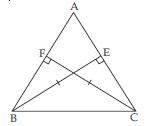In $∆$ABE and $∆$ACF,
∠A = ∠A (Common)
∠AEB = ∠AEC (each = 90$°$)
[$\because$ BE $\perp$ AC and CF $\perp$AB (given)]
BE = CF (given)
(i) $\therefore$ $∆$ABE $\cong$ $∆$ACF
[AAS criteria of congruency]
(ii) So, AB = AC
[Congruent parts of congruent triangles]
i.e., $∆$ABC is an isosceles triangle.

##### Question 17:

ABC and DBC are two isosceles triangles on the same base BC (see fig.). Show that ∠ABD = ∠ACD.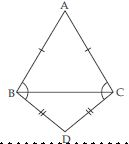In isosceles $∆$ABC,
AB = AC (given)
$\therefore$ ∠ACB = ∠ABC ... (i)
(angles opposite to equal sides)
Also in isosceles $∆$BCD,
BD = DC
$\therefore$ ∠BCD = ∠CBD ... (ii)
(angles opposite to equal sides)
Adding corresponding sides of (i) and (ii),
∠ACB + ∠BCD = ∠ABC + ∠CBD
$⇒$ ∠ACD = ∠ABD
or ∠ABD = ∠ACD [Hence Proved]

##### Question 18:

$∆$ABC is an isosceles triangle in which AB = AC. Side BA is produced to D such that AD = AB (see fig.). Show that ∠BCD is a right angle.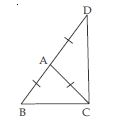In an isosceles triangle ABC;
AB = AC
$\therefore$ ∠ACB = ∠ABC ... (i)
(angles opposite to equal sides)
Now AD = AB (By construction)
But AB = AC (given)
$\therefore$ AD = AB = AC
$⇒$ AD = AC
Now in $∆$ADC,
$⇒$ ∠ADC = ∠ACD ... (ii)
[angles opposite to equal sides of $∆$ADC]
From Fig., we have
∠BAC + ∠CAD = 180$°$ ... (iii)
(linear pair)
As we know that exterior angle of a triangle is equal to the sum of its interior opposite angles.
$\therefore$ In $∆$ABC,
= ∠ACB + ∠ACB [Using (i)]
$⇒$ ∠CAD = 2∠ACB ... (iv)
Similarly for $∆$ADC;
[Same reason as above]
= ∠ACD + ∠ACD[Using (ii)]
$⇒$ ∠BAC = 2∠ACD ... (v)
From (iii), (iv) and (v), we get:
2∠ACB + 2∠ACD=180$°$
or 2(∠ACB + ∠ACD)=180$°$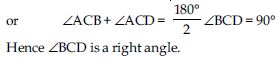##### Question 19:

ABC is a right angled triangle in which ∠A = 90$°$ and AB = AC. Find ∠B and ∠C.

ABC is a right triangle in which
∠A = 90$°$
and AB = AC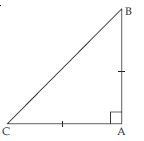In $∆$ABC,
AB = AC
$⇒$ ∠C = ∠B ... (i)
(angles opposite to equal sides)
Now in $∆$ABC,
∠A + ∠B + ∠C = 180$°$
(angles sum property)
$⇒$ 90$°$ + ∠B + ∠B = 180$°$
[$\because$ ∠A = 90$°$ (Given) and ∠B = ∠C (from (i)]
$⇒$ 2∠B = 180$°$ – 90$°$
$⇒$ 2∠B = 90$°$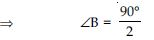$⇒$ ∠B = 45$°$
Also ∠C = ∠B
$⇒$ ∠C = 45$°$

##### Question 20:

Show that the angles of an equilateral triangle are 60$°$ each.

Let ABC be an equilateral triangle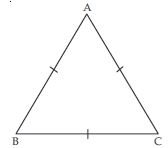$\therefore$ AB = BC = AC
AB = BC
$⇒$ ∠C = ∠A .. (i)
(angles opp. to equal sides)
Similarly
AB = AC
$⇒$ ∠C = ∠B ... (ii)
(angles opp. to equal sides)
From (i) and (ii), we get
∠A = ∠B = ∠C ... (iii)
Now in $∆$ABC;
∠A + ∠B + ∠C= 180$°$ ... (iv)
[angles sum property]
$⇒$ ∠A + ∠A + ∠A=180$°$
$⇒$ 3∠A=180º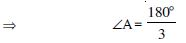$⇒$ ∠A=60$°$
From (iii); ∠A = ∠B = ∠C
$⇒$ ∠A = ∠B = ∠C = 60$°$
Hence each angle of an equilateral triangle is 60$°$.

##### Question 21:

$∆$ABC and $∆$DBC are two isosceles triangles on the same base BC and vertices A and D are on the same side of BC (see fig.). If AD is extended to intersect BC at P, show that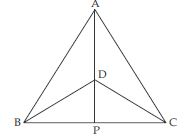$∆$ABD $\cong$ $∆$ACD

$∆$ABC is an isosceles triangle.
$\therefore$ AB = AC
$∆$DBC is also an isosceles triangle.
$\therefore$ BD = CD
Now in $∆$ABD and $∆$ACD
AB = AC (given)
BD = CD (given)
$∆$ABD $\cong$ $∆$ACD
(SSS criteria of congruency)
Proved part (i)
$⇒$ ∠BAD = ∠CAD ... (a)
[Congruent parts of congruent triangles]

##### Question 22:

$∆$ABC and $∆$DBC are two isosceles triangles on the same base BC and vertices A and D are on the same side of BC (see fig.). If AD is extended to intersect BC at P, show that$∆$ABP $\cong$ $∆$ACP

$∆$ABC is an isosceles triangle.
$\therefore$ AB = AC
$∆$DBC is also an isosceles triangle.
$\therefore$ BD = CD
Now in $∆$ABD and $∆$ACD
AB = AC (given)
BD = CD (given)
Now in $∆$ABP and $∆$ACP
AB = AC (given)
AP = AP (common)
$\therefore$ $∆$ABP $\cong$ $∆$ACP
(SAS criteria of congruency)

##### Question 23:

$∆$ABC and $∆$DBC are two isosceles triangles on the same base BC and vertices A and D are on the same side of BC (see fig.). If AD is extended to intersect BC at P, show thatAP bisects ∠A as well as ∠D.

$∆$ABC is an isosceles triangle.
$\therefore$ AB = AC
$∆$DBC is also an isosceles triangle.
$\therefore$ BD = CD
Now in $∆$ABD and $∆$ACD
AB = AC (given)
BD = CD (given)
In part (ii), we have proved that
$∆$ABP $\cong$ $∆$ACP
So, ∠BAP = ∠CAP
(Congruent parts of congruent triangles)
It implies that AP bisects ∠A.
In part (i) we have proved that
$∆$ABD $\cong$ $∆$ACD
(Congruent parts of congruent triangles)
∠ADB + ∠BDP = 180$°$
(linear pair) ... (c)
∠ADC + ∠CDP = 180$°$ ... (d)
(linear pair)
From (c) and (d), we get:
[Using (b)]
$⇒$ ∠BDP = ∠CDP
$⇒$ DP bisects ∠D.
or we can say that AP bisects ∠D.

##### Question 24:

$∆$ABC and $∆$DBC are two isosceles triangles on the same base BC and vertices A and D are on the same side of BC (see fig.). If AD is extended to intersect BC at P, show thatAP is the perpendicular bisector of BC.

$∆$ABC is an isosceles triangle.
$\therefore$ AB = AC
$∆$DBC is also an isosceles triangle.
$\therefore$ BD = CD
Now in $∆$ABD and $∆$ACD
AB = AC (given)
BD = CD (given)
In part (ii) we have proved that
$∆$ABP $\cong$ $∆$ACP
$\therefore$ BP = PC ...(e)
(Congruent parts of congruent triangles)
and ∠APB = ∠APC (c.p.c.t.) ... (f)
Now ∠APB + ∠APC = 180$°$ (linear pair)
$⇒$ ∠APB + ∠APB = 180$°$ [Using (f)]
$⇒$ 2∠APB = 180$°$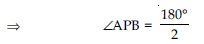$⇒$ ∠APB = 90$°$
$⇒$ AP $\perp$ BC ... (g)
From (e) we have BP = PC and from (g), we have
proved AP $\perp$ BC. So, collectively we can say that
AP is perpendicular bisector of BC.

##### Question 25:

AD is an altitude of an isosceles triangle ABC in which AB = AC. Show that

In $∆$ABD and $∆$ACD,
AB = AC (given)
∠ADB = ∠ADC (each = 90$°$)
[$\because$ AD $\perp$ BC (given)]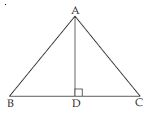$\therefore$ $∆$ABD $\cong$ $∆$ACD
(RHS rule of congruency)
So, BD = DC
(Congruent parts of congruent triangles)
It implies AD; bisects BC. (Proved part (i))
(Congruent parts of congruent triangles)

##### Question 26:

Two sides AB and BC and median AM of one triangle ABC are respectively equal to sides PQ and QR and median PN of $∆$PQR (see fig). Show that: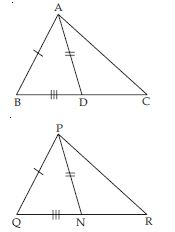$∆$ABM $\cong$ $∆$PQNNow in $∆$ABM and $∆$PQN,
AB = PQ (given)
AM = PN (given)
BM = QN [proved in part (c)]
$\therefore$ $∆$ABM $\cong$ $∆$PQN
(SSS criteria of congruency)
So, ∠B = ∠Q ... (d)
(Congruent parts of congruent triangles)

##### Question 27:

Two sides AB and BC and median AM of one triangle ABC are respectively equal to sides PQ and QR and median PN of $∆$PQR (see fig). Show that:$∆$ABC $\cong$ $∆$PQRIn $∆$ABC and $∆$PQR,
AB = PQ (given)
∠B = ∠Q [Using part (d)]
BC = QR (given)
$\therefore$ $∆$ABC $\cong$ $∆$PQR
(SAS criteria of congruency)

##### Question 28:

BE and CF are two equal altitudes of a triangle ABC. Using RHS congruence rule, prove that the triangle ABC is isosceles.

In $∆$BEC and $∆$CFB,
∠BEC = ∠CFB (each = 90$°$)
[$\because$ BE $\perp$ AC and CF $\perp$ AB]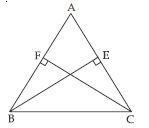BC = BC (common)
BE = CF (given)
$\therefore$ $∆$BEC $\cong$ $∆$CFB (RHS rule of congruency)
So, EC = FB ...(i)
(Congruent parts of congruent triangles)
Now, in $∆$AEB and $∆$AFC,
∠A = ∠A (common)
∠AEB = ∠AFC
(each = 90$°$) [given]
EB = FC (given)
$\therefore$ $∆$AEB $\cong$ $∆$AFC
(AAS criteria of congruency)
So, AE = AF ...(ii)
(Congruent parts of congruent triangles)
Adding (i) and (ii), we get:
EC + AE= FB + AF
$⇒$ AC = AB
Now, in $∆$ABC; we have
AB = AC
It implies that $∆$ABC is isosceles.

##### Question 29:

ABC is an isosceles triangle with AB = AC. Draw AP $\perp$ BC and show that ∠B = ∠C.

Given: ABC is an isosceles triangle in which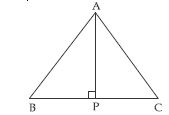AB=AC
To prove: ∠B=∠C
Construction: Draw AP $\perp$ BC.
Proof: In $∆$ABP and $∆$ACP,
∠APB=∠APC
(each = 90$°$) [By construction]
AB =AC (given)
AP=AP (common)
$\therefore$ $∆$ABP$\cong$ $∆$ACP
(RHS rule of congruency)
So, ∠B=∠C
(Congruent parts of congruent triangles)

##### Question 30:

Show that in a right angled triangle, the hypotenuse is the longest side.

Let ABC be a right angled triangle, rt. angle at B. We have to prove that AC, the hypotenuse is the longest side.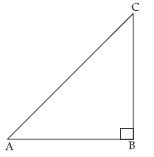In rt. $∆$ABC;
$⇒$ ∠A + ∠B + ∠C= 180$°$
∠A + 90$°$+ ∠C = 180$°$ [Since, ∠B = 90$°$]
$⇒$ ∠A + ∠C= 180$°$ – 90$°$ = 90$°$
Now ∠A + ∠C= 90$°$
and ∠B= 90$°$
$⇒$ ∠B >∠C and ∠B > ∠A
As we know that the greater angle has a longer
side opposite to it.
AC > AB and AC > BC.
In other words, ∠B being the greatest angle has the longest opposite side AC i.e., hypotenuse.

##### Question 31:

In fig., sides AB and AC of $∆$ABC are extended to points P and Q respectively. Also, ∠PBC < ∠QCB. Show that AC> AB.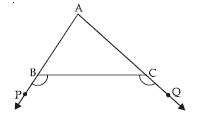∠ABC + ∠PBC = 108$°$ ...(i)
(linear pair axiom)
∠ACB + ∠QCB = 180$°$ ...(ii)
(linear pair axiom)
From (i) and (ii), we get:
∠ABC + ∠PBC = ∠ACB + ∠QCB ...(iii)
But ∠PBC, ∠QCB (given) ...(iv)
$\therefore$ Using (iv) in (iii), we have
∠ABC > ∠ACB
Now in $∆$ABC
∠ABC > ∠ACB
[Proved above in (iv)]
$\therefore$ AC > AB
[Since, Side opposite to greater angle is longer.]

##### Question 32:

In fig., ∠B < ∠A and ∠C < ∠D. Show that AD < BC.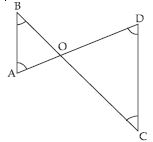In $∆$AOB;
∠B < ∠A (given)
or ∠A > ∠B
So; OB > OA ...(i)
[Since, Side opposite to greater angle is longer]
In $∆$ COD;
∠C < ∠D (given)
or ∠D > ∠C
$\therefore$ OC > OD ...(ii)
[Since, Side opposite to greater angle is longer]
Adding (i) and (ii), we get:
OB + OC > OA + OD
$⇒$ BC > AD
or AD < BC [Hence Proved]

##### Question 33:

AB and CD are respectively the smallest and longest sides of a quadrilateral ABCD (see fig., ). Show that ∠A >∠C and ∠B > ∠D.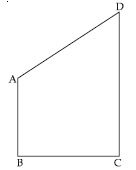Given: ABCD is a quadrilateral. AB is the shortest side and CD is the longest side.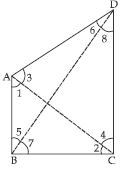To prove: ∠A > ∠C and ∠B > ∠D.
Construction: Join A and C also B and D.
Proof: In $∆$ABC, AB is the smallest side
$\therefore$ BC > AB
$⇒$ ∠1 > ∠2 ...(i)
[Since, Angle opposite to longer side is greater]
In $∆$ADC, CD is the largest side
$\therefore$ CD > AD
$⇒$ ∠3 > ∠4 ...(ii)
Adding (i) and (ii), we get
∠1 + ∠3 > ∠2 + ∠4
$⇒$ ∠A >∠C [This proves 1st part]
Now in $∆$ADB, AB is the smallest side
$\therefore$ AD > AB
$⇒$ ∠5 > ∠6 ...(iii)
and in $∆$BCD, CD is the greatest side
$\therefore$ CD > BC $⇒$ ∠7 > ∠8 ...(iv)
Adding (iii) and (iv), we get:
∠5 + ∠7 > ∠6 + ∠8
∠B > ∠D [This proves 2nd part]
Hence ∠A > ∠C and ∠B > ∠D
[Hence Proved]

##### Question 34:

In fig. PR >PQ and PS bisects ∠QPR. Prove that ∠PSR > ∠PSQ.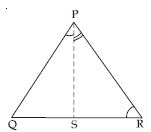In $∆$PQR,
PR > PQ (given)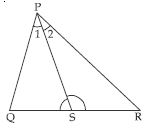$\therefore$ ∠PQR > ∠PRQ
(∠opp. to longer side is greater) ...(1)
Again ∠1 = ∠2
[Since, PS is the bisector of ∠P] ...(2)
$\therefore$ ∠PQR + ∠1 > ∠PRQ + ∠2 ...(3)
But ∠PQS + ∠1 + ∠PSQ =
∠PRS + ∠2 + ∠PSR = 180$°$
[Sum of ∠s of $∆$ is 180$°$]
$\therefore$ ∠PQR + ∠1 + ∠PSQ =
∠PRQ + ∠2 + ∠PSR ...(4)
[Since ∠PRS = ∠PRQ and
∠PQS = ∠PQR]
From (3) and (4), we get
∠PSQ < ∠PSR
or ∠PSR > ∠PSQ

##### Question 35:

Show that of all line segments drawn from a given point not on it, the perpendicular line segment is the shortest.

Given: l is a line and P is point not lying on l.
PM $\perp$ l.
N is any point on l other than M.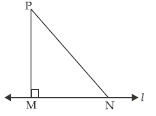To prove: PM < PN
Proof: In $∆$PMN, ∠M is the right angle. So, N is an acute angle.
(Angle sum property of triangle)
$\therefore$ ∠M > ∠N.
$\therefore$ PN > PM (Side opp. greater angle)
or PM < PN.
Hence, of all line segments drawn from a given point not on it, the perpendicular is the shortest.

##### Question 36:

ABC is a triangle. Locate a point in the interior of $∆$ ABC which is equidistant from all the vertices of $∆$ABC.

Let ABC be a triangle.
Draw perpendicular bisectors PQ and RS of sides AB and BC respectively of triangle ABC.
Let PQ bisects AB at M and RS bisects BC at point N.
Let PQ and RS intersect at point O.
Join OA, OB and OC.
Now in $∆$AOM and BOM
AM = MB (By construction)
∠AMO = ∠BMO
(each = 90$°$) [By construction]
OM = OM (Common)
$\therefore$ $∆$AOM $\cong$ $∆$BOM
(SAS criteria of congruency)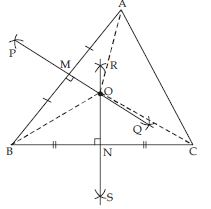So, OA = OB (c.p.c.t.) ....(i)
Similarly, $∆$BON $\cong$ $∆$CON
$⇒$ OB = OC (c.p.c.t) ....(ii)
From (i) and (ii), we observe that
OA = OB = OC
Hence, O is the point of intersection of perpendicular bisectors of any two sides of $∆$ABC equidistant from its vertices.

##### Question 37:

In a triangle locate a point in its interior which is equidistant from all the sides of the triangle.

Let ABC be a triangle.
Draw bisectors of ∠B and ∠C.
Let these angle bisectors intersect each other at
point I.
Draw IK $\perp$ BC
Also draw IJ $\perp$ AB
and IL $\perp$ AC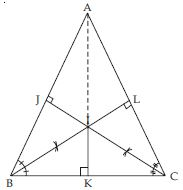Join AI.
In $∆$BIK and $∆$BIJ,
∠IKB = ∠IJB (each = 90$°$)
(By construction)
∠IBK = ∠IBJ
[$\because$ BI is the bisector of ∠B]
(By construction)
BI = BI (Common)
$\therefore$ $∆$BIK $\cong$ $∆$BIJ
(AAS criteria of congruency)
$\therefore$ IK = IJ
(Congruent parts of congruent triangles) ....(i)
Similarly, $∆$CIK $\cong$ $∆$CIL
So IK = IL (c.p.c.t) ....(ii)
From (i) and (ii), we get:
IJ = IK = IL ;
Hence, I is the point of intersection of angle bisectors of any two angles of $∆$ABC equidistant from its sides.

##### Question 38:

In a huge park, people are concentrated at three points (see fig.).
A: where there are different slides and swings for children.
B: near which a man-made lake is situated.
C: which is near to a large parking and exit.
Where should an icecream parlour be set up so that maximum number of persons can approach it?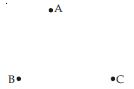The parlour should be equidistant from A, B
and C.
For this let we draw perpendicular bisector say l of line joining points B and C also draw perpendicular bisector say m of line joining points A and C.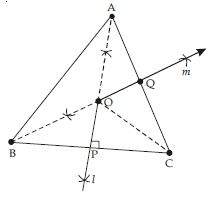Let l and m intersect each other at point O.
Now point O is equidistant from points A, B and C.
Join OA, OB and OC.
Proof: In $∆$BOP and $∆$COP
OP = OP (Common)
∠OPB = ∠OPC (each = 90$°$)
(By Const.)
BP = PC
[$\because$ P is the mid-point of BC]
$\therefore$ $∆$BOP $\cong$ $∆$COP
[SAS criteria of congruency]
So OB = OC (c.p.c.t.) ...(i)
Similarly $∆$AOQ $\cong$ $∆$COQ
$⇒$ OA = OC (c.p.c.t.) ..(ii)
From (i) and (ii), we get:
OA = OB = OC
We observe that ice-cream parlour should be set up at point O; the point of intersection of perpendicular bisectors of any two sides out of three formed by joining these points.

##### Question 39:

Complete the hexagonal and star shaped Rangolies (see figs.) by filling them with as many equilateral triangles of side 1 cm as you can. Count the number of triangles in each case. Which has more triangles?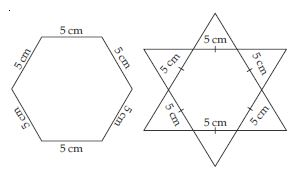In hexagonal rangoli: Number of equilateral triangles each of side 5 cm = 6
$\therefore$ Area of equilateral triangle of side 5 cm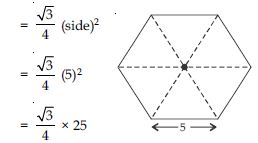Area of hexagonal rangoli = 6 × area of an equilateral triangle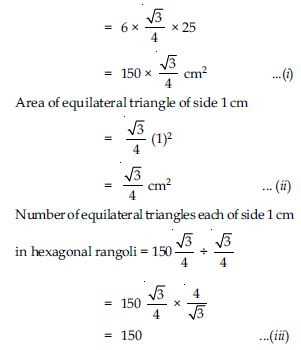Now in Star Rangoli
Number of equilateral triangles each of side 5 cm = 12
So, total area of star rangoli = 12 × area of an equilateral triangle of side 5 cm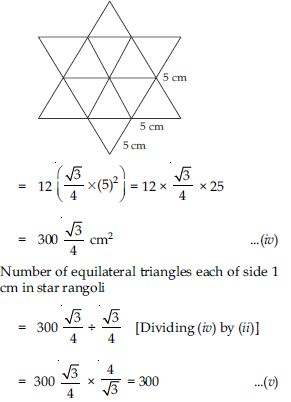From (iii) and (v), we observe that star rangoli has more equilateral triangles each of side 1 cm.